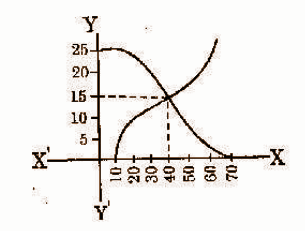# Andhra Pradesh SSC Board Question Paper for Class 10th Maths Paper 2 2017 In PDF

## AP SSC or 10th Class Question Paper Mathematics Paper 2 English Medium 2017 with Solutions – Free Download

Andhra Pradesh SSC (Class 10) Maths 2017 question paper 2 with solutions are available here in a downloadable pdf format and also in the text so that the students can easily obtain them. Along with the solutions, they can also get the Maths question paper 2 2017 Class 10 SSC for reference. All the Andhra Pradesh board previous year Maths question papers are available here. AP 10th Class Mathematics Question Paper 2017 Paper 2 can be downloaded easily and students can understand the concept, solve problems and verify the answers provided by BYJU’S. Solving 2017 Maths question paper 2 for Class 10 will help the students to predict the type of questions that will appear in the exam.

### QUESTION PAPER CODE 16E(A)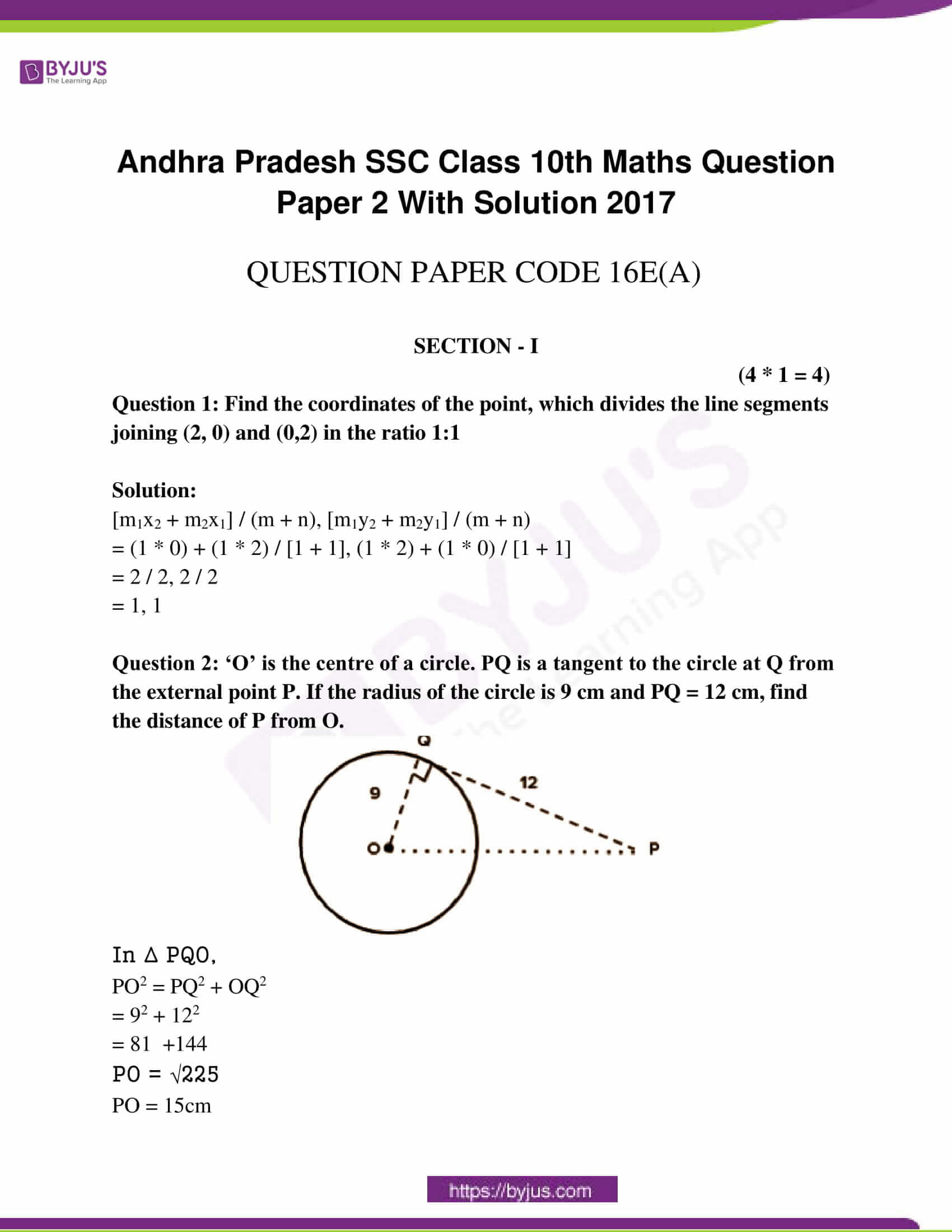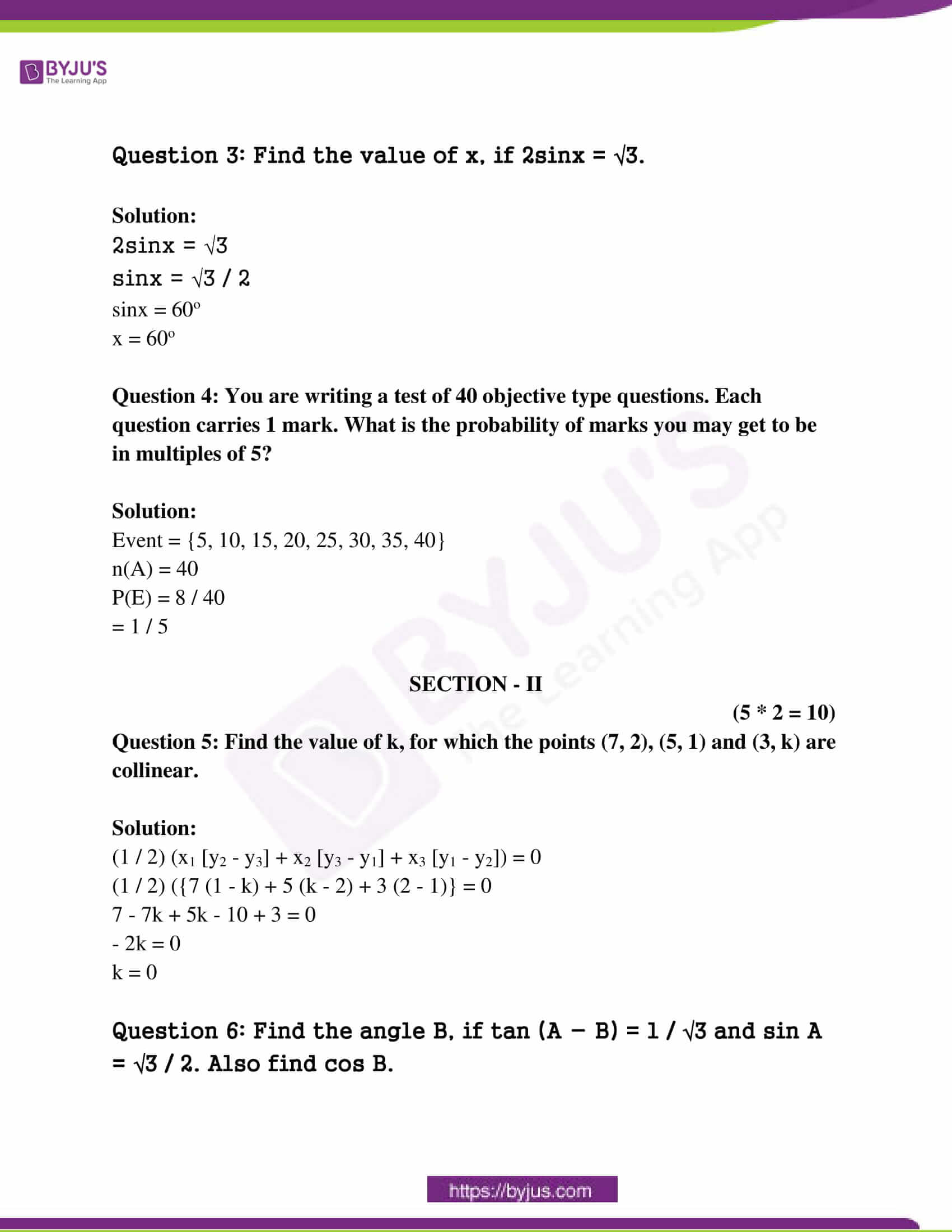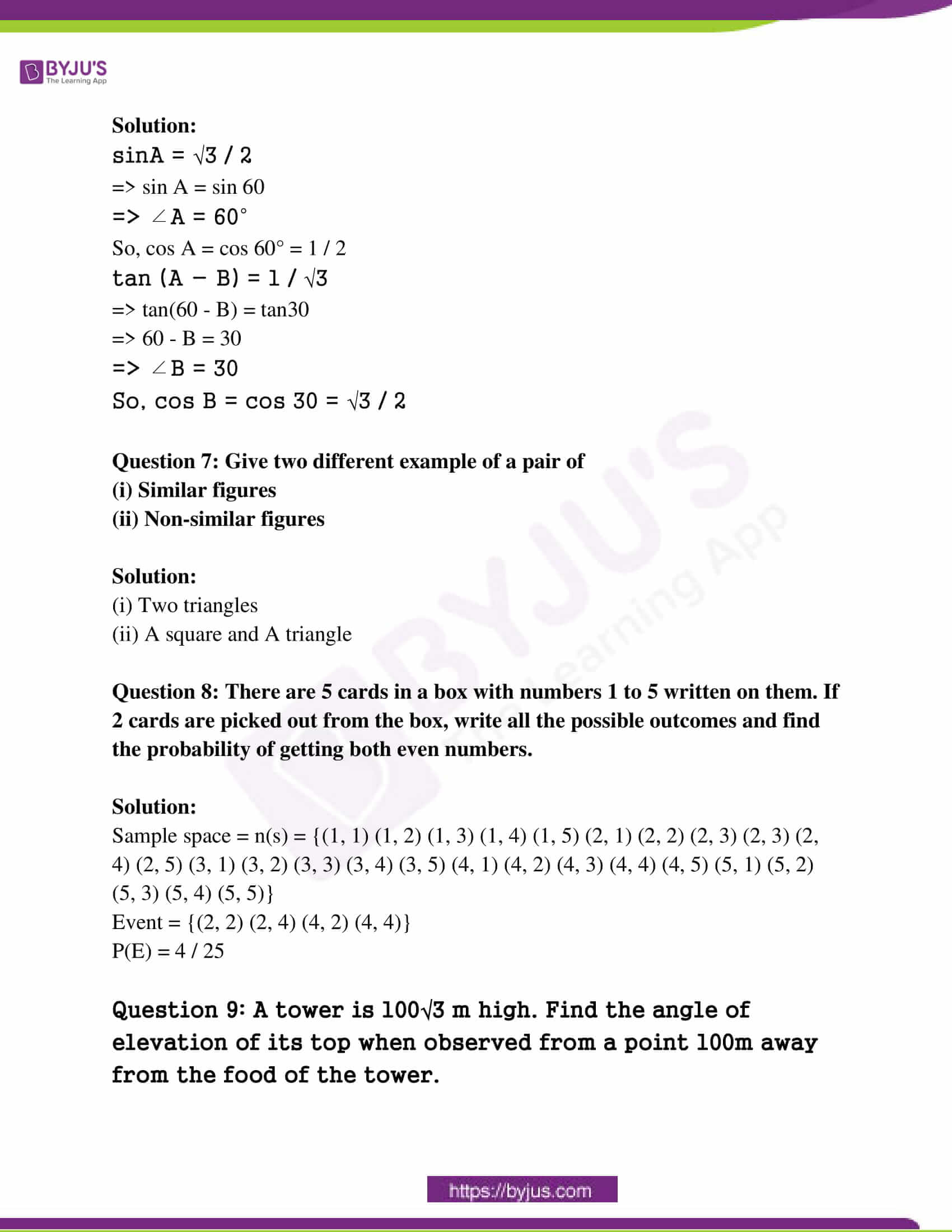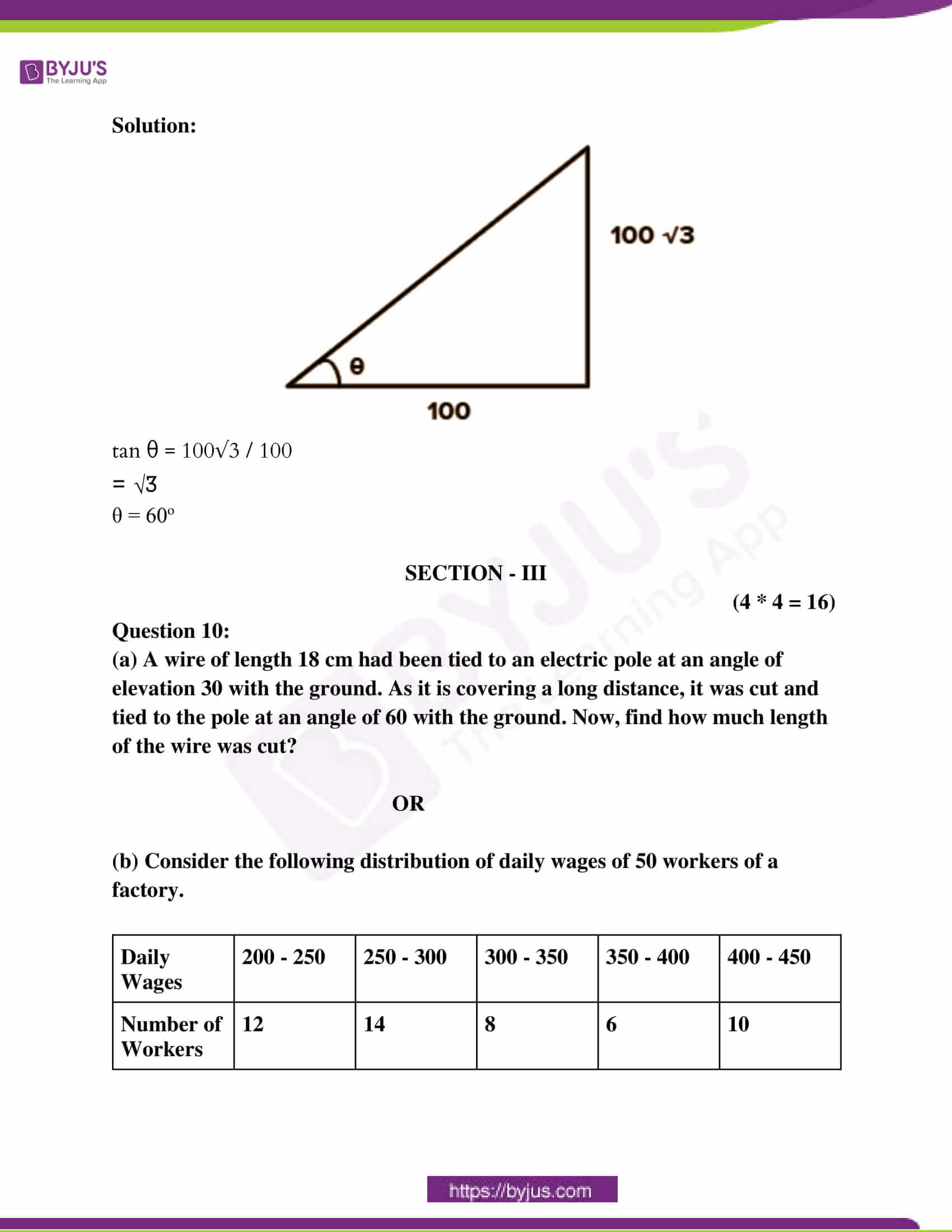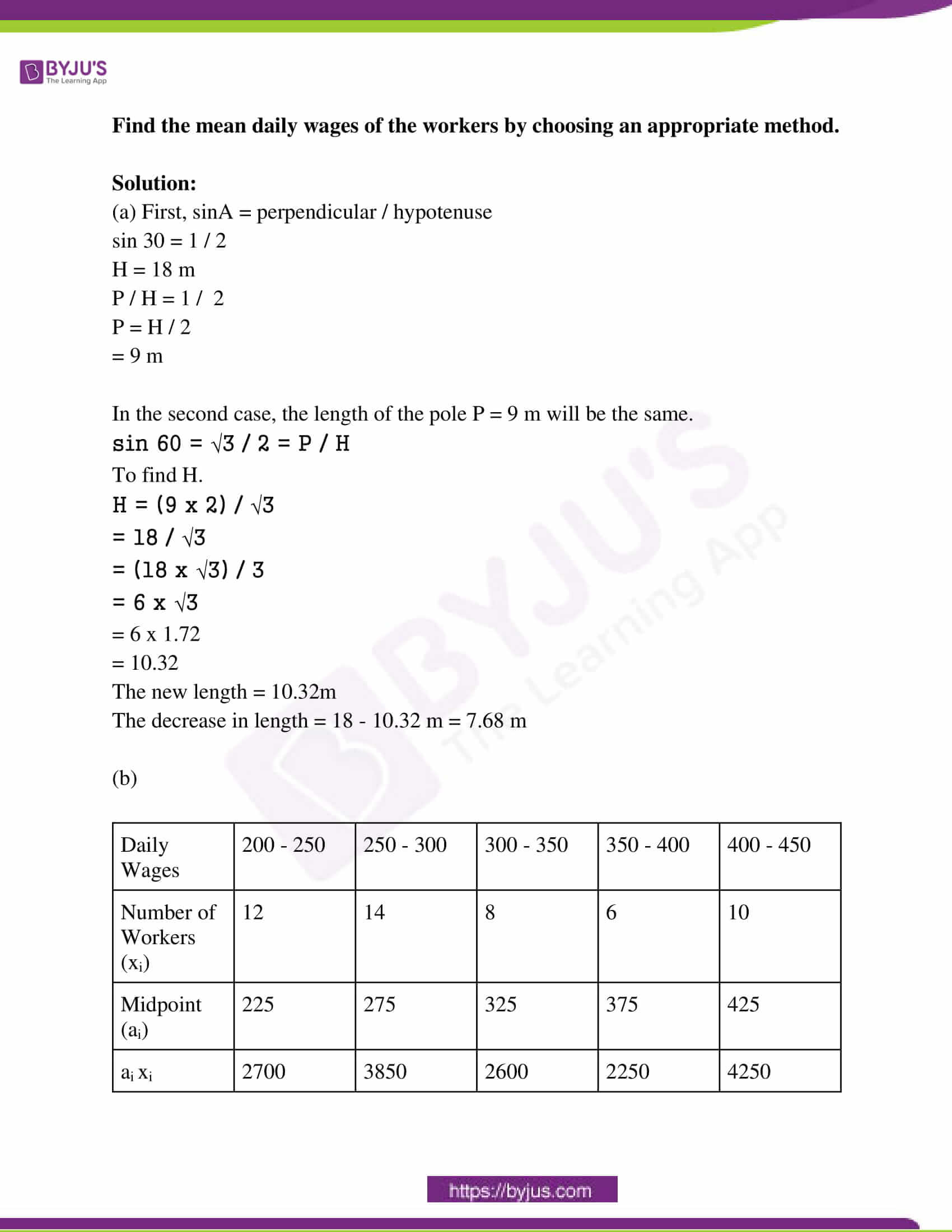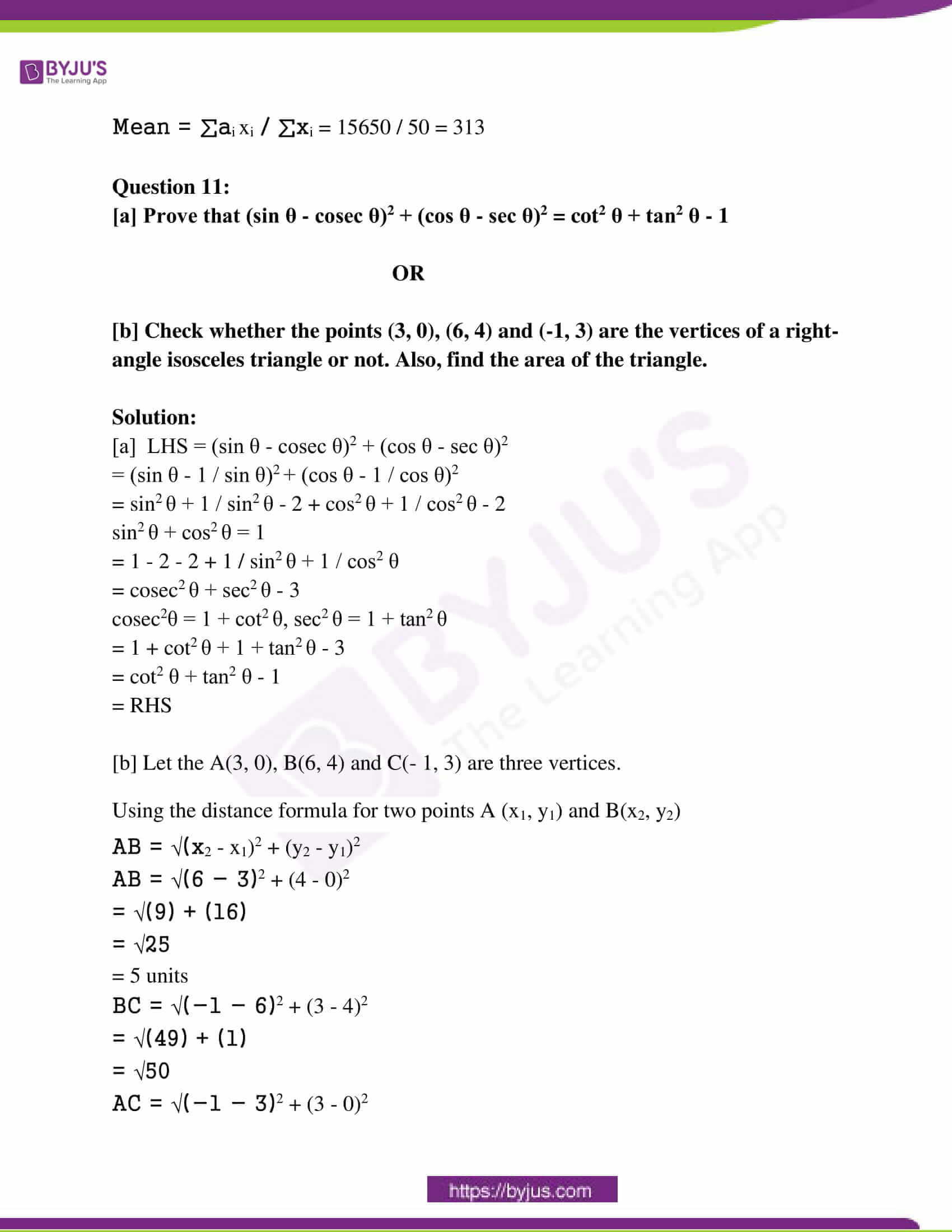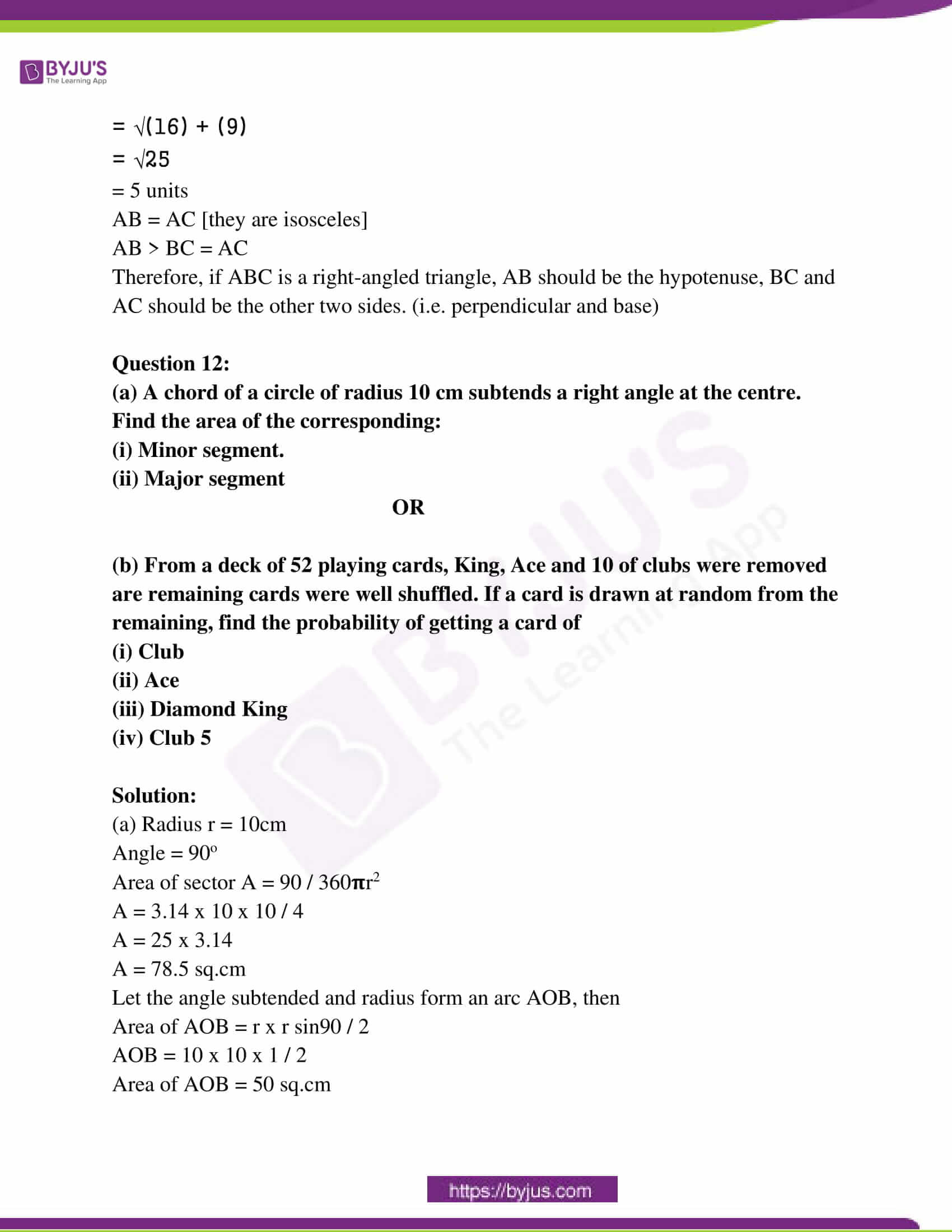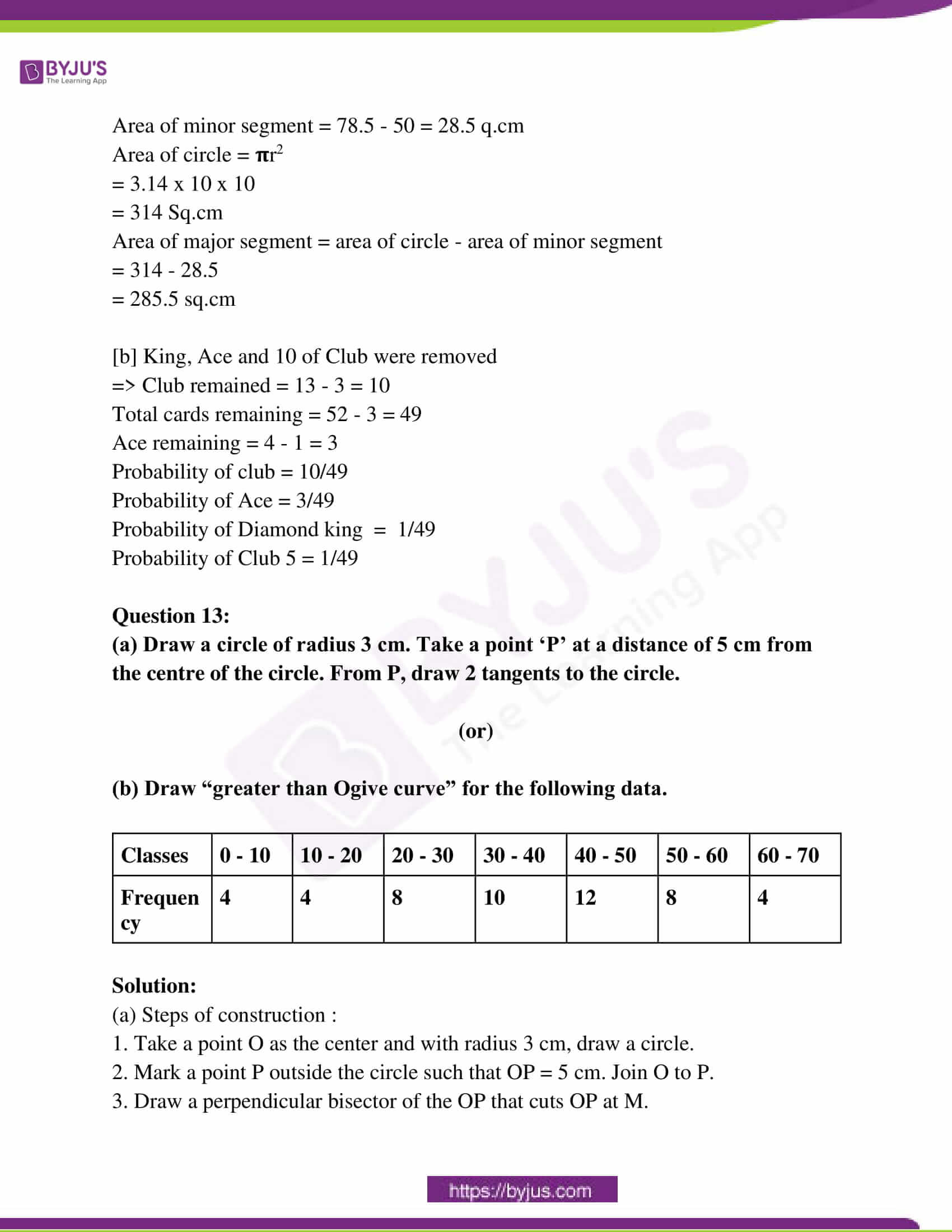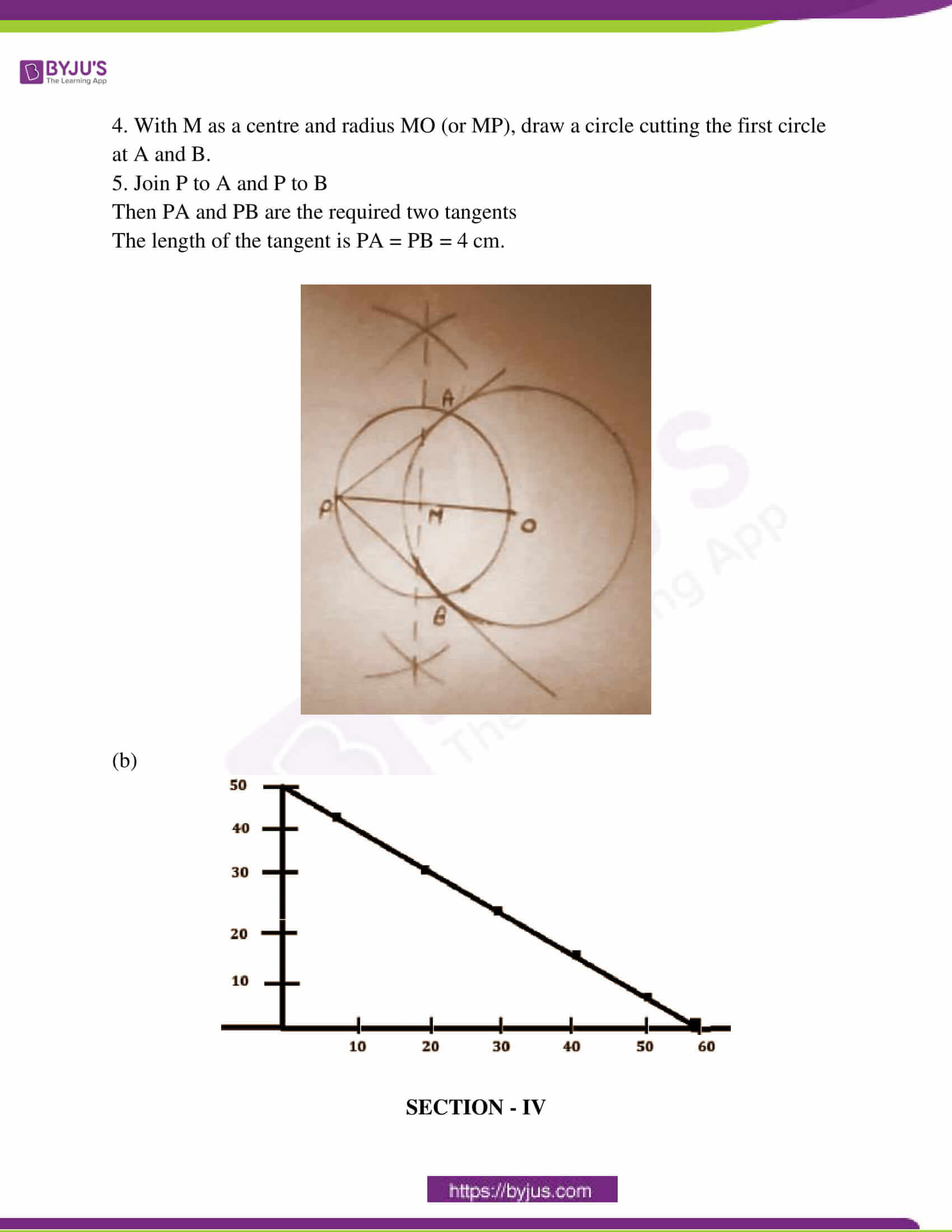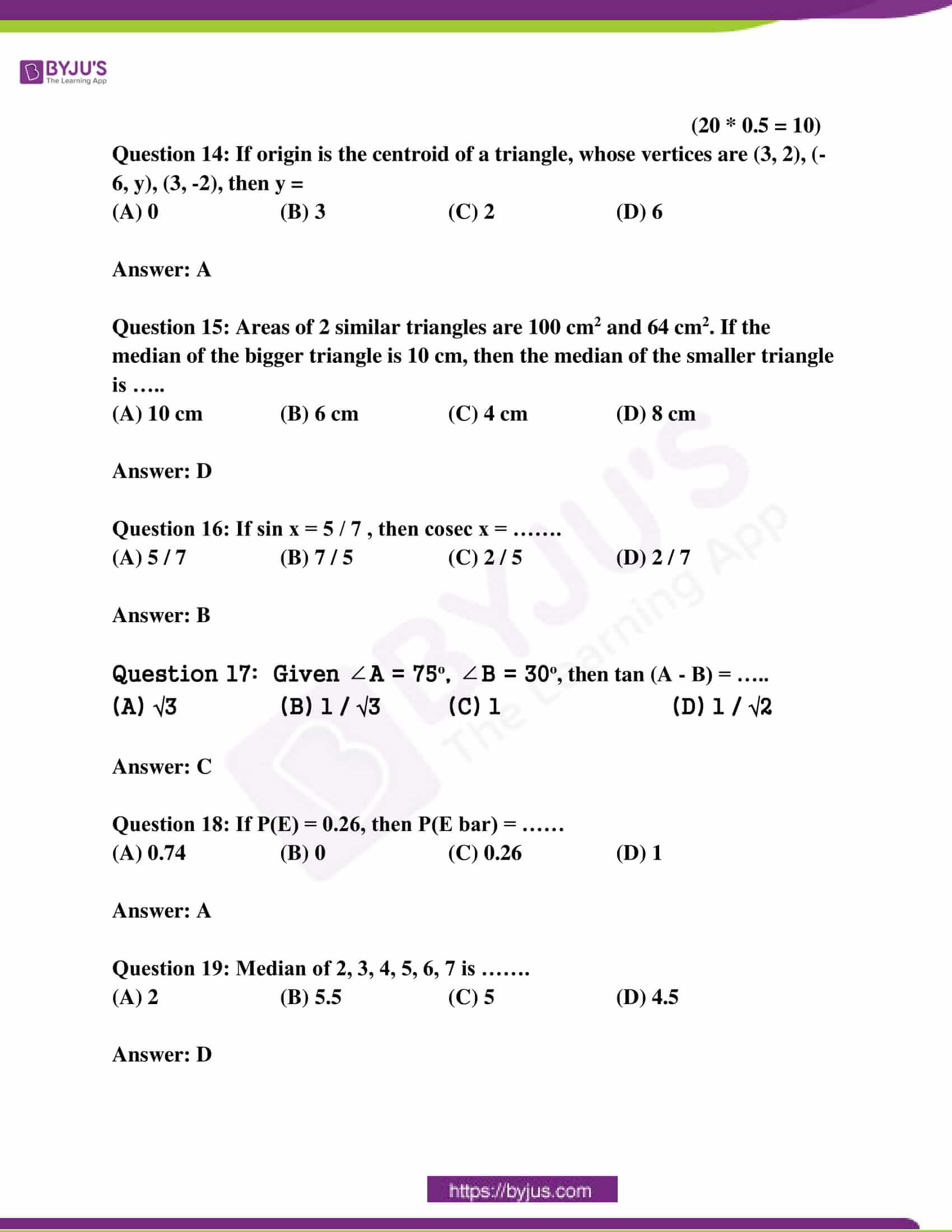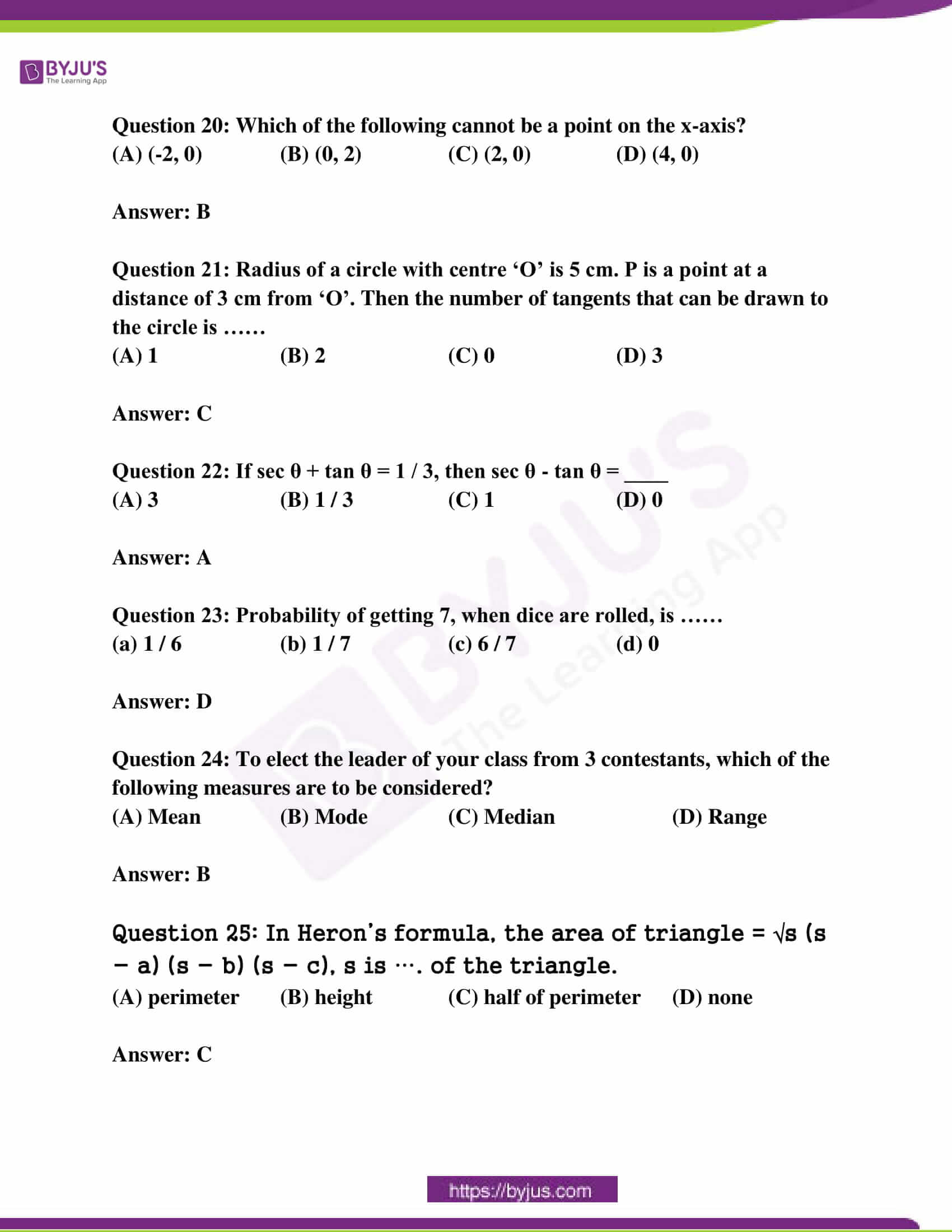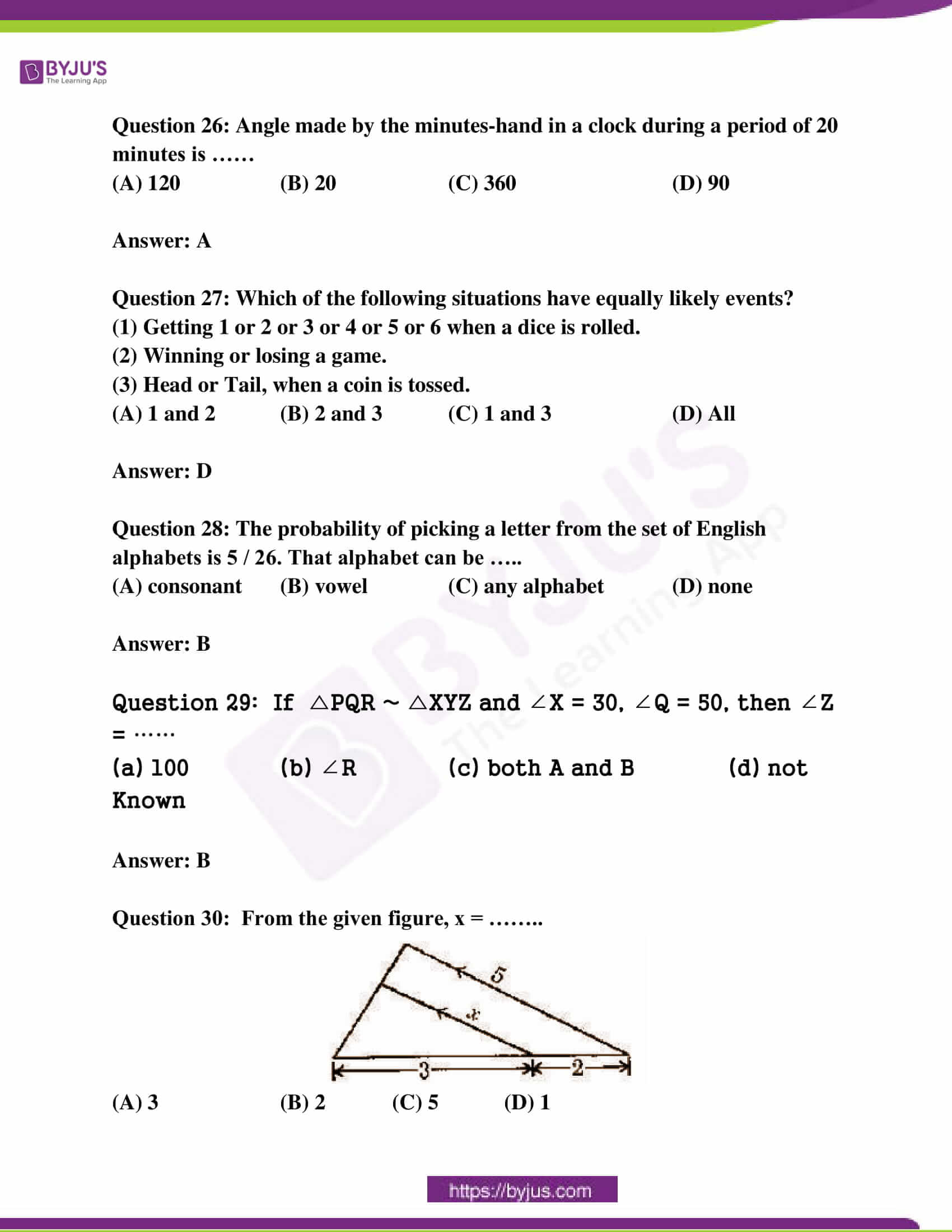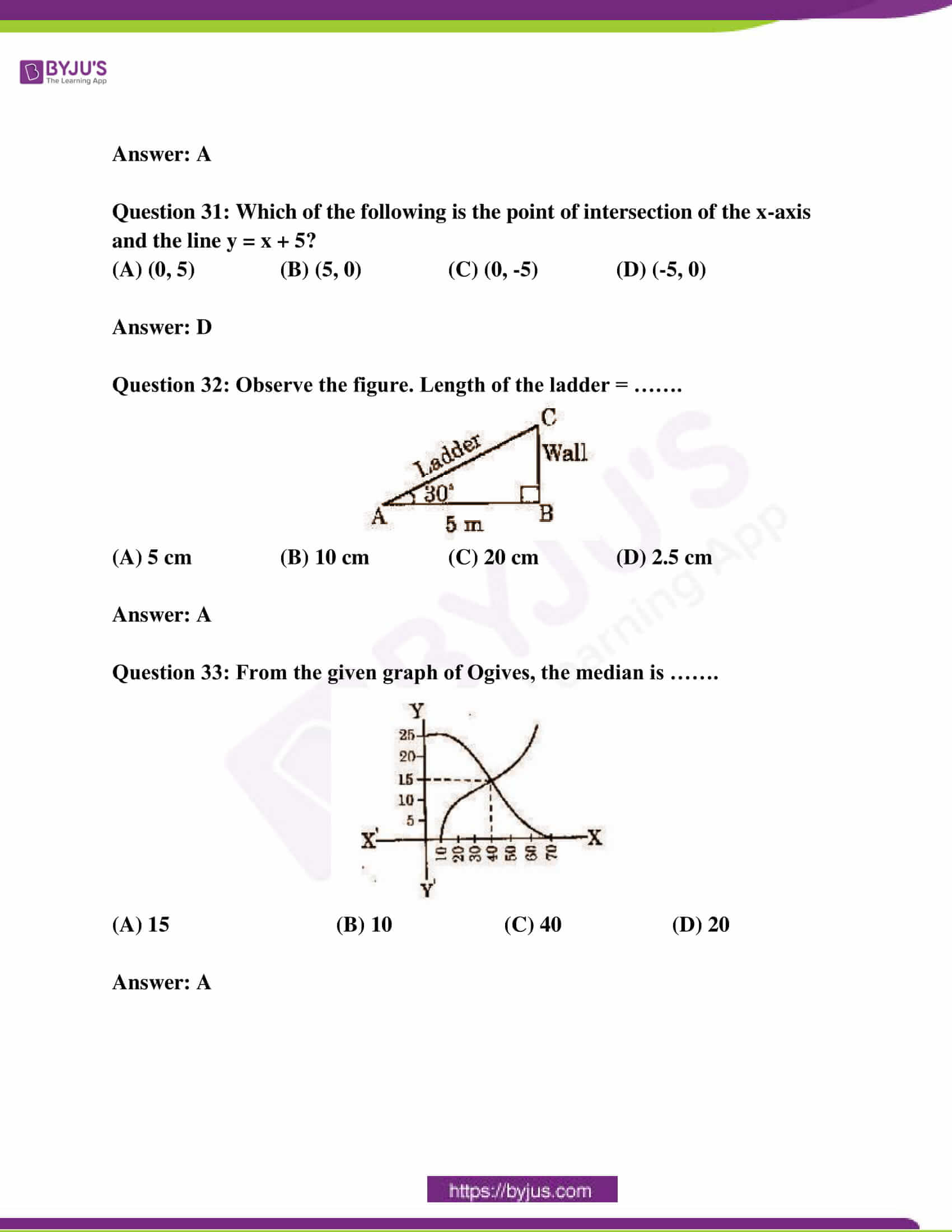SECTION – I

(4 * 1 = 4)

Question 1: Find the coordinates of the point, which divides the line segments joining (2, 0) and (0,2) in the ratio 1:1

Solution:

[m1x2 + m2x1] / (m + n), [m1y2 + m2y1] / (m + n)

= (1 * 0) + (1 * 2) / [1 + 1], (1 * 2) + (1 * 0) / [1 + 1]

= 2 / 2, 2 / 2

= 1, 1

Question 2: ‘O’ is the centre of a circle. PQ is a tangent to the circle at Q from the external point P. If the radius of the circle is 9 cm and PQ = 12 cm, find the distance of P from O.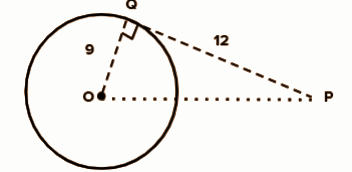In ∆ PQO,

PO2 = PQ2 + OQ2

= 92 + 122

= 81 +144

PO = √225

PO = 15cm

Question 3: Find the value of x, if 2sinx = √3.

Solution:

2sinx = √3

sinx = √3 / 2

sinx = 60o

x = 60o

Question 4: You are writing a test of 40 objective type questions. Each question carries 1 mark. What is the probability of marks you may get to be in multiples of 5?

Solution:

Event = {5, 10, 15, 20, 25, 30, 35, 40}

n(A) = 40

P(E) = 8 / 40

= 1 / 5

SECTION – II

(5 * 2 = 10)

Question 5: Find the value of k, for which the points (7, 2), (5, 1) and (3, k) are collinear.

Solution:

(1 / 2) (x1 [y2 – y3] + x2 [y3 – y1] + x3 [y1 – y2]) = 0

(1 / 2) ({7 (1 – k) + 5 (k – 2) + 3 (2 – 1)} = 0

7 – 7k + 5k – 10 + 3 = 0

– 2k = 0

k = 0

Question 6: Find the angle B, if tan (A – B) = 1 / √3 and sin A = √3 / 2. Also find cos B.

Solution:

sinA = √3 / 2

=> sin A = sin 60

=> ∠A = 60°

So, cos A = cos 60° = 1 / 2

tan (A – B) = 1 / √3

=> tan(60 – B) = tan30

=> 60 – B = 30

=> ∠B = 30

So, cos B = cos 30 = √3 / 2

Question 7: Give two different examples of a pair of

(i) Similar figures

(ii) Non-similar figures

Solution:

(i) Two triangles

(ii) A square and A triangle

Question 8: There are 5 cards in a box with numbers 1 to 5 written on them. If 2 cards are picked out from the box, write all the possible outcomes and find the probability of getting both even numbers.

Solution:

Sample space = n(s) = {(1, 1) (1, 2) (1, 3) (1, 4) (1, 5) (2, 1) (2, 2) (2, 3) (2, 3) (2, 4) (2, 5) (3, 1) (3, 2) (3, 3) (3, 4) (3, 5) (4, 1) (4, 2) (4, 3) (4, 4) (4, 5) (5, 1) (5, 2) (5, 3) (5, 4) (5, 5)}

Event = {(2, 2) (2, 4) (4, 2) (4, 4)}

P(E) = 4 / 25

Question 9: A tower is 100√3 m high. Find the angle of elevation of its top when observed from a point 100m away from the food of the tower.

Solution: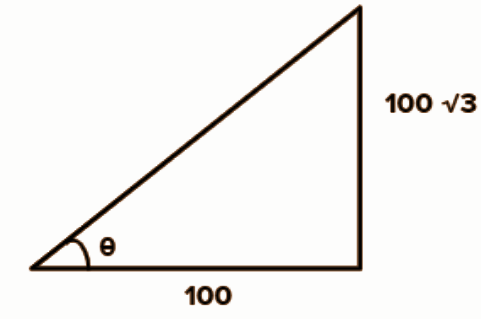tan θ = 100√3 / 100

= √3

θ = 60o

SECTION – III

(4 * 4 = 16)

Question 10:

(a) A wire of length 18 cm had been tied to an electric pole at an angle of elevation 30 with the ground. As it is covering a long distance, it was cut and tied to the pole at an angle of 60 with the ground. Now, find how much length of the wire was cut?

OR

(b) Consider the following distribution of daily wages of 50 workers of a factory.

 Daily Wages 200 – 250 250 – 300 300 – 350 350 – 400 400 – 450 Number of Workers 12 14 8 6 10

Find the mean daily wages of the workers by choosing an appropriate method.

Solution:

(a) First, sinA = perpendicular / hypotenuse

sin 30 = 1 / 2

H = 18 m

P / H = 1 / 2

P = H / 2

= 9 m

In the second case, the length of the pole P = 9 m will be the same.

sin 60 = √3 / 2 = P / H

To find H.

H = (9 x 2) / √3

= 18 / √3

= (18 x √3) / 3

= 6 x √3

= 6 x 1.72

= 10.32

The new length = 10.32m

The decrease in length = 18 – 10.32 m = 7.68 m

(b)

 Daily Wages 200 – 250 250 – 300 300 – 350 350 – 400 400 – 450 Number of Workers (xi) 12 14 8 6 10 Midpoint (ai) 225 275 325 375 425 ai xi 2700 3850 2600 2250 4250

Mean = ∑ai xi / ∑xi = 15650 / 50 = 313

Question 11:

[a] Prove that (sin θ – cosec θ)2 + (cos θ – sec θ)2 = cot2 θ + tan2 θ – 1

OR

[b] Check whether the points (3, 0), (6, 4) and (-1, 3) are the vertices of a right-angle isosceles triangle or not. Also, find the area of the triangle.

Solution:

[a] LHS = (sin θ – cosec θ)2 + (cos θ – sec θ)2

= (sin θ – 1 / sin θ)2 + (cos θ – 1 / cos θ)2

= sin2 θ + 1 / sin2 θ – 2 + cos2 θ + 1 / cos2 θ – 2

sin2 θ + cos2 θ = 1

= 1 – 2 – 2 + 1 / sin2 θ + 1 / cos2 θ

= cosec2 θ + sec2 θ – 3

cosec2θ = 1 + cot2 θ, sec2 θ = 1 + tan2 θ

= 1 + cot2 θ + 1 + tan2 θ – 3

= cot2 θ + tan2 θ – 1

= RHS

[b] Let the A(3, 0), B(6, 4) and C(- 1, 3) are three vertices.

Using the distance formula for two points A (x1, y1) and B(x2, y2)

AB = √(x2 – x1)2 + (y2 – y1)2

AB = √(6 – 3)2 + (4 – 0)2

= √(9) + (16)

= √25

= 5 units

BC = √(-1 – 6)2 + (3 – 4)2

= √(49) + (1)

= √50

AC = √(-1 – 3)2 + (3 – 0)2

= √(16) + (9)

= √25

= 5 units

AB = AC [they are isosceles]

AB > BC = AC

Therefore, if ABC is a right-angled triangle, AB should be the hypotenuse, BC and AC should be the other two sides. (i.e. perpendicular and base)

Question 12:

(a) A chord of a circle of radius 10 cm subtends a right angle at the centre. Find the area of the corresponding:

(i) Minor segment.

(ii) Major segment

OR

(b) From a deck of 52 playing cards, King, Ace and 10 of clubs were removed and the remaining cards were well shuffled. If a card is drawn at random from the remaining, find the probability of getting a card of

(i) Club

(ii) Ace

(iii) Diamond King

(iv) Club 5

Solution:

Angle = 90o

Area of sector A = 90 / 360𝛑r2

A = 3.14 x 10 x 10 / 4

A = 25 x 3.14

A = 78.5 sq.cm

Let the angle subtended and radius form an arc AOB, then

Area of AOB = r x r sin90 / 2

AOB = 10 x 10 x 1 / 2

Area of AOB = 50 sq.cm

Area of minor segment = 78.5 – 50 = 28.5 q.cm

Area of circle = 𝛑r2

= 3.14 x 10 x 10

= 314 Sq.cm

Area of major segment = area of circle – area of minor segment

= 314 – 28.5

= 285.5 sq.cm

[b] King, Ace and 10 of Club were removed

=> Club remained = 13 – 3 = 10

Total cards remaining = 52 – 3 = 49

Ace remaining = 4 – 1 = 3

Probability of club = 10/49

Probability of Ace = 3/49

Probability of Diamond king = 1/49

Probability of Club 5 = 1/49

Question 13:

(a) Draw a circle of radius 3 cm. Take a point ‘P’ at a distance of 5 cm from the centre of the circle. From P, draw 2 tangents to the circle.

(or)

(b) Draw “greater than Ogive curve” for the following data.

 Classes 0 – 10 10 – 20 20 – 30 30 – 40 40 – 50 50 – 60 60 – 70 Frequency 4 4 8 10 12 8 4

Solution:

(a) Steps of construction :

1. Take a point O as the center and with radius 3 cm, draw a circle.

2. Mark a point P outside the circle such that OP = 5 cm. Join O to P.

3. Draw a perpendicular bisector of the OP that cuts OP at M.

4. With M as a centre and radius MO (or MP), draw a circle cutting the first circle at A and B.

5. Join P to A and P to B

Then PA and PB are the required two tangents

The length of the tangent is PA = PB = 4 cm.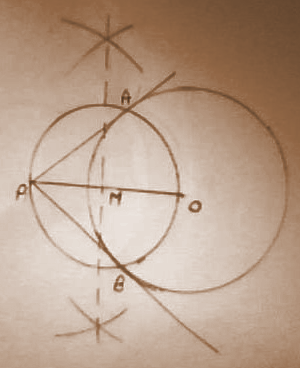(b)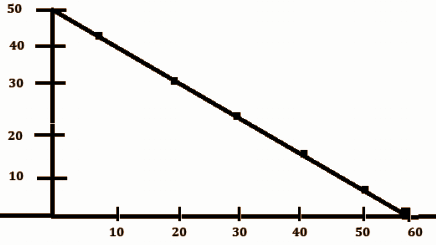SECTION – IV

(20 * 0.5 = 10)

Question 14: If origin is the centroid of a triangle, whose vertices are (3, 2), (-6, y), (3, -2), then y =

(A) 0 (B) 3 (C) 2 (D) 6

Question 15: Areas of 2 similar triangles are 100 cm2 and 64 cm2. If the median of the bigger triangle is 10 cm, then the median of the smaller triangle is …..

(A) 10 cm (B) 6 cm (C) 4 cm (D) 8 cm

Question 16: If sin x = 5 / 7 , then cosec x = …….

(A) 5 / 7 (B) 7 / 5 (C) 2 / 5 (D) 2 / 7

Question 17: Given ∠A = 75o, ∠B = 30o, then tan (A – B) = …..

(A) √3 (B) 1 / √3 (C) 1 (D) 1 / √2

Question 18: If P(E) = 0.26, then P(E bar) = ……

(A) 0.74 (B) 0 (C) 0.26 (D) 1

Question 19: Median of 2, 3, 4, 5, 6, 7 is …….

(A) 2 (B) 5.5 (C) 5 (D) 4.5

Question 20: Which of the following cannot be a point on the x-axis?

(A) (-2, 0) (B) (0, 2) (C) (2, 0) (D) (4, 0)

Question 21: Radius of a circle with centre ‘O’ is 5 cm. P is a point at a distance of 3 cm from ‘O’. Then the number of tangents that can be drawn to the circle is ……

(A) 1 (B) 2 (C) 0 (D) 3

Question 22: If sec θ + tan θ = 1 / 3, then sec θ – tan θ = ____

(A) 3 (B) 1 / 3 (C) 1 (D) 0

Question 23: Probability of getting 7, when dice are rolled, is ……

(a) 1 / 6 (b) 1 / 7 (c) 6 / 7 (d) 0

Question 24: To elect the leader of your class from 3 contestants, which of the following measures are to be considered?

(A) Mean (B) Mode (C) Median (D) Range

Question 25: In Heron’s formula, the area of triangle = √s (s – a) (s – b) (s – c), s is …. of the triangle.

(A) perimeter (B) height (C) half of perimeter (D) none

Question 26: Angle made by the minutes-hand in a clock during a period of 20 minutes is ……

(A) 120 (B) 20 (C) 360 (D) 90

Question 27: Which of the following situations have equally likely events?

(1) Getting 1 or 2 or 3 or 4 or 5 or 6 when a dice is rolled.

(2) Winning or losing a game.

(3) Head or Tail, when a coin is tossed.

(A) 1 and 2 (B) 2 and 3 (C) 1 and 3 (D) All

Question 28: The probability of picking a letter from the set of English alphabets is 5 / 26. That alphabet can be …..

(A) consonant (B) vowel (C) any alphabet (D) none

Question 29: If △PQR ~ △XYZ and ∠X = 30, ∠Q = 50, then ∠Z = ……

(a) 100 (b) ∠R (c) both A and B (d) not Known

Question 30: From the given figure, x = ……..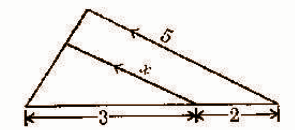(A) 3 (B) 2 (C) 5 (D) 1

Question 31: Which of the following is the point of intersection of the x-axis and the line y = x + 5?

(A) (0, 5) (B) (5, 0) (C) (0, -5) (D) (-5, 0)

Question 32: Observe the figure. Length of the ladder = …….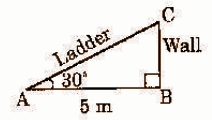(A) 5 cm (B) 10 cm (C) 20 cm (D) 2.5 cm